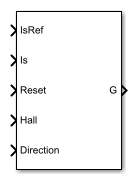# BLDC Current Controller with PWM Generation

Discrete-time brushless DC Motor current PI controller with pulse width modulation generation

•Libraries:
Simscape / Electrical / Control / BLDC Control

## Description

The BLDC Current Controller with PWM Generation block generates a pulse width modulation (PWM) signal and controls current in a brushless DC motor. The controller uses this algorithm.### Equations

The BLDC Current Controller with PWM Generation produces the duty cycle for a BLDC block by implementing proportional-integral (PI) current control using this equation

`$\text{D}=\left({K}_{p}+{K}_{i}\frac{{T}_{s}z}{z-1}\right)\left({I}_{s_ref}-{I}_{s}\right)$`

where:

• D is the duty cycle.

• Kp is the proportional gain.

• Ki is the integral gain.

• Ts is the time period.

• Is_ref is the reference current.

• Is is the measured current.

• Gzc is the zero cancellation polynomial.

The closed-loop transfer function for the PI control algorithm yields a zero that can be cancelled by using zero-cancellation block in the feedforward path. The zero-cancellation transfer function in discrete-time is:

`${G}_{ZC}\left(z\right)=\frac{\frac{{T}_{s}{K}_{i}}{{K}_{p}}}{z+\left(\frac{{T}_{s}-\frac{{K}_{p}}{{K}_{i}}}{\frac{{K}_{p}}{{K}_{i}}}\right)}.$`

The block obtains control signals for the three phases by multiplying the duty cycle by the commutation signals. The resulting three control signals are normalized over the interval [-1, 1].

The PWM generator outputs a 1 when the value of the control signal is greater than the carrier counter value. Otherwise, the PWM generator outputs a 0.

## Ports

### Input

expand all

Reference current for control.

Data Types: `single` | `double`

Actual current.

Data Types: `single` | `double`

External reset signal (rising edge) for the integrator.

Data Types: `single` | `double`

Hall sensor data.

Data Types: `single` | `double`

Direction of motor rotation.

Data Types: `single` | `double`

### Output

expand all

Pulse waveforms that determine switching behavior in the attached block.

Data Types: `single` | `double`

## Parameters

expand all

### Control Parameters

Proportional gain, Kp, of the controller.

Integral gain, Ki, of the controller.

Anti-windup gain, Kaw, of the controller.

Time, in s, between consecutive block executions. During execution, the block produces outputs and, if appropriate, updates its internal state. For more information, see What Is Sample Time? and Specify Sample Time.

If this block is inside a triggered subsystem, inherit the sample time by setting this parameter to `-1`. If this block is in a continuous variable-step model, specify the sample time explicitly using a positive scalar.

#### Dependencies

If you set Sample time (-1 for inherited) to `-1` and select the Enable zero cancellation option, the Discretization sample time parameter becomes visible.

Time, in s, between consecutive discretizations. Discretization is required for zero cancellation.

#### Dependencies

This parameter is only visible when both these conditions are met:

• is set to `-1`.

• Enable zero cancellation is selected.

Option to use zero cancellation on the feedforward path.

#### Dependencies

If you select the Enable zero cancellation option and set Sample time (-1 for inherited) to `-1`, the Discretization sample time parameter becomes visible.

### PWM Generator

Use the carrier counter strategy to change the initial behavior of the PWM output:

• Up counter — PWM output begins at the start of the on state.

• Down counter — PWM output begins at the start of the off state.

• Up-down counter — PWM output begins in the middle of the on state.

Pulse width modulation timer period, Tper, in seconds.

Time, in s, between consecutive PWM generator executions. During execution, the block produces PWM output and, if appropriate, updates its internal state. For more information, see What Is Sample Time? and Specify Sample Time.

To ensure adequate resolution in the generated PWM signal, set the fundamental sample time so that $0<{T}_{s\text{_}pwm}\le 10{T}_{per}$, where:

• Ts_pwm is the Fundamental sample time (s).

• Tper is the Timer period (s).

 Stirban, A., I. Boldea, and G. D. Andreescu. "Motion-Sensorless Control of BLDC-PM Motor With Offline FEM-Information-Assisted Position and Speed Observer." IEEE Transactions on Industry Applications. 48, no. 6 (2012): 1950-1958.